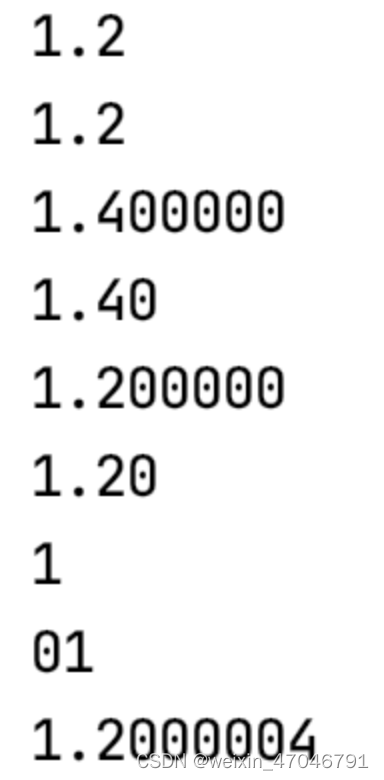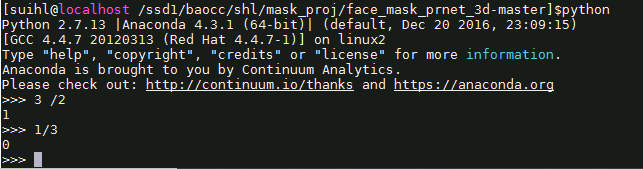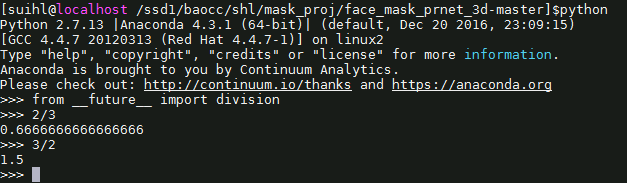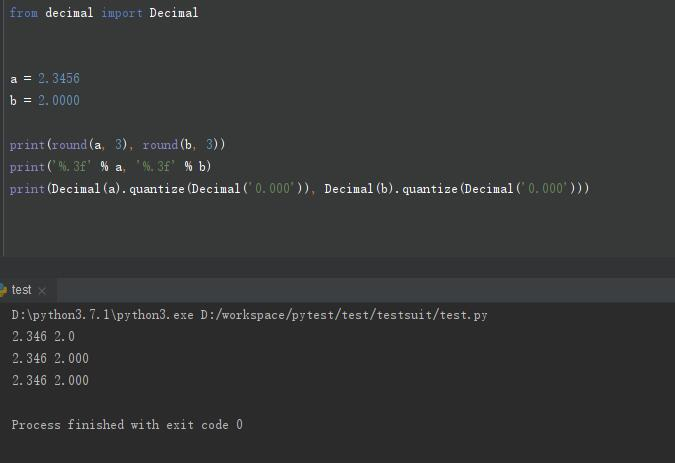• 2021-04-08 20:22:51

python保留小数位的三种方法

保留小数位是我们经常会碰到的问题，尤其是刷题过程中。那么在python中保留小数位的方法也非常多，但是笔者的原则就是什么简单用什么，因此这里介绍几种比较简单实用的保留小数位的方法：

方法一：format函数

>>> print('{:.3f}'.format(1.23456))
1.235
>>> print(format(1.23456, '.2f'))
1.23


方法二：’%.xf’方法

>>> print('%.2f' % 1.23456)
1.23


方法三：round()函数


>>> print(round(1.23456,3))
1.235
>>> print(round(2.355,2))
2.35
>>> print(round(2.5))
2
>>> print(round(3.5))
4


注意：

round()函数比较迷，它不完全是按照四舍五入的原则来进位的，因此不建议大家使用。它的保留值将保留到离上一位更近的一端（四舍六入），如果距离两端一样远，则会保留到偶数的一边。好了笔者也要绕晕了，反正笔者是不会用这种方法的。

更多相关内容
• 那么在python保留小数位的方法也非常多，但是笔者的原则就是什么简单用什么，因此这里介绍几种比较简单实用的保留小数位的方法： 方法一：format函数 >>> print('{:.3f}'.format(1.23456)) 1.235 >>> print...
• 一：使用占位符方法： # 1：%g 科学计数法...使用%.2f 表示保留位小数 print("%f"%(1.4)) print("%.2f"%(1.4)) print("%f"%(1.2000004)) print("%.2f"%(1.2000004)) # 3.%d保留整数 print("%d"%(1.2000004)) pri

一：使用占位符方法：

# 1：%g 科学计数法输出小数，用于舍弃无效的小数
print("%g"%(1.2000004))
print("%.2g"%(1.2000004))
# 2：直接使用%f 输出小数，会出现无效的0；使用%.2f 表示保留两位小数
print("%f"%(1.4))
print("%.2f"%(1.4))
print("%f"%(1.2000004))
print("%.2f"%(1.2000004))
# 3.%d保留整数
print("%d"%(1.2000004))
print("%.2d"%(1.2000004))
# 4.%s一般表示字符串占位
print("%s"%(1.2000004))

输出结果：二：round方法

print(round(2.342343, 2))

三：实战（要求将数字转化字符串并显示千分符号且保留2位小数）

format(num,",") 其中num表示数字类型，不能使用字符串
num=32468762348.87
#先保留两位小数
str_num="%.2f"%(num)
#将str_num类型转成float类型
result_num=format(float(str_num),",")
print(result_num)   #32,468,762,348.87

四：当遇到整数时，转化字符串并显示千分符号且保留2位小数

用上述方法只能保留一位小数，此时需要做一个判断

num=763246872
str_num="%.2f"%(num)
result_num=format(float(num),",")
if len(result_num.split(".")[-1])<2:
result_num=result_num+"0"
print(result_num)   #763,246,872.00

展开全文开发语言
• python保存两位小数的几种方法文章目录：一、保留位小数 且 做四舍五入处理1、使用字符串格式化2、使用python内置的round() 函数3、使用python内置的decimal模块二、保留位小数 且 不做四舍五入处理1、使用序列...

# 一、保留两位小数 且 做四舍五入处理

## 1、使用字符串格式化


>>> x = 3.1415926
>>> print("%.2f" % x)
3.14
>>>


## 2、使用python内置的round() 函数

>>> x = 3.1415926
>>> round(x, 2)
3.14
>>>


round()函数的官方定义：

def round(number, ndigits=None): # real signature unknown; restored from __doc__
"""
round(number[, ndigits]) -> number

Round a number to a given precision in decimal digits (default 0 digits).
This returns an int when called with one argument, otherwise the
same type as the number. ndigits may be negative.
"""
return 0


## 3、使用python内置的decimal模块

decimal 英 /'desɪm(ə)l/ 小数的
quantize 英 /'kwɒntaɪz/ 量化

>>> from decimal import Decimal
>>> x = 3.1415926
>>> Decimal(x).quantize(Decimal("0.00"))
Decimal('3.14')
>>> a = Decimal(x).quantize(Decimal("0.00"))
>>> print(a)
3.14
>>> type(a)
<class 'decimal.Decimal'>
>>> b = str(a)
>>> b
'3.14'


# 二、保留两位小数 且 不做四舍五入处理

## 1、使用序列中的切片

>>> x = 3.1415926
>>> str(x).split(".") + "." + str(x).split(".")[:2]
'3.14'


## 2、使用re正则匹配模块

>>> import re
>>> x = 3.1415926
>>> re.findall(r"\d{1,}?\.\d{2}", str(a))
['3.14']


# 三、python2保留小数

1、python2中除法，默认是取商，也就是在做除法的时候你是无法获取小数部分的！

如下：2、解决方法，就是在脚本文件中开头导入未来版本功能，如下：

from __future__ import division
import os

print(2/3)注意：

from __future__ import division一定要在其他模块之前导入，否则报错！♠ ⊕ ♠ ⊕ ♠ ⊕ ♠ ⊕ ♠ ⊕ ♠ ⊕ ♠ ⊕ ♠ ⊕ ♠ ⊕ ♠ ⊕ ♠ ⊕ ♠ ⊕ ♠ ⊕ ♠ ⊕ ♠ ⊕ ♠ ⊕ ♠ ⊕ ♠ ⊕ ♠ ⊕ ♠ ⊕ ♠ ⊕ ♠ ⊕ ♠ ⊕ ♠ ⊕ ♠ ⊕ ♠ ⊕ ♠ ⊕ ♠ ⊕ ♠ ⊕ ♠ ⊕ ♠

展开全文• 转自：Python 保留n位小数 输出a, b 且保留三位小数 a = 2.3456 b = 2.0000 三种方法:round(a, 3)'%.3f' % aDecimal(a).quantize(Decimal('0.000')) 转自：Python 保留n位小数

输出a, b 且保留三位小数

a = 2.3456
b = 2.0000

三种方法:

round(a, 3)
'%.3f' % a
Decimal(a).quantize(Decimal('0.000'))展开全文java tensorflow
• Python 保留小数1.可以使用format内置函数格式化输出import mathPI = math.atan(1.0)*4 # atan(1.0)*4 = πprint(’{:.7f}’.format(PI))结果：3.14159272.使用 ‘%’import mathPI = math.atan(1.0)*4print(’%.7f...
• round(a,2)=12.35 保留三位小数 方法2， 例如： a=12.34567889 print（“%.2f”%a) 保留两位小数 print（“%.3f”%a) 保留三位小数 print（“%.4f”%a) 保留四位小数 方法3， 首先引入decima函数 fr...
• ## Python中保留两位小数的几种方法

万次阅读 多人点赞 2018-12-14 21:43:57
保留位小数，并做四舍五入处理 方法一: 使用字符串格式化 &gt;&gt;&gt; a = 12.345 &gt;&gt;&gt; print("%.2f" % a) 12.35 &gt;&gt;&gt; 方法二: 使用round内置...Decimal 字符串格式化
• 前言 在对数据进行处理时我们常常会用到format与round函数。二者都能保留若干位小数，但在处理过程... 位数保留不同：规定如保留6位小数，format函数输出结果小数点后面就是保留6位小数，而round函数稍微灵活点，它会开发语言 后端
• ## Python保留指定位数的小数

万次阅读 多人点赞 2019-09-06 10:22:51
Python保留指定位数的小数1 ’%.2f’ %f 方法(推荐)2 format函数(推荐)3 round（）函数4 不进行四舍五入，直接进行截断 1 ’%.2f’ %f 方法(推荐) ﻿﻿f = 1.23456 print('%.4f' % f) print('%.3f' % f) print('%....
• 以上这篇python 除法保留小数点的方法就是小编分享给大家的全部内容了，希望能给大家一个参考，也希望大家多多支持软件开发网。 您可能感兴趣的文章:浅谈Python里面小数点精度的控制pandas 小数位数 精度的处理...
• ## python三种保留两位小数的方法

万次阅读 多人点赞 2020-12-25 17:03:03
python三保留位小数方法汇总 #’%.2f’%f #format函数 #round函数 1.’%.2f’%f 该方法会进行四舍五入 下面展示一些 内联代码片。 f = 2.3456789 print('%.2f'%f) print('%.3f'%f) print('%.4f'%f) 结果如下所...编程语言
• #保留三位小数截断 python3 print(int(1.23456 * 1000) / 1000 ) (2) 使用字符串截取，截取小数点后指定的位数 AI悦创·推出辅导班啦，包括「Python 语言辅导班、C++ 辅导班、java 辅导班、算法/数据结构辅导班、...开发语言
• Python取整及保留小数 欢迎使用Markdown编辑器 你好！ 这是你第一次使用 Markdown编辑器 所展示的欢迎页。如果你想学习如何使用Markdown编辑器, 可以仔细阅读这篇文章，了解一下Markdown的基本语法知识。 新的改变 ...
• ## Python保留n位小数

千次阅读 2019-06-13 18:43:00
输出a, b 且保留三位小数 a = 2.3456 b = 2.0000 三种方法:round(a, 3)('%.3f' % a)Decimal(a).quantize(Decimal('0.000') 转载于:https://www.cnblogs.com/xioawu-blog/p/11018687.html
• python输入保存两位小数的四种办法：a = 5.5461办法一：round(a,2)办法二：float('%.2f' % a)相干保举：《Python视频教程》办法：‘%.2’ %a办法四：from decimal import DecimalDecimal('5.026').quantize...
• 保留位小数，并做四舍五入处理 方法一: 使用字符串格式化 方法二: 使用round内置函数 方法: 使用decimal模块 仅保留位小数，无需四舍五入 方法一: 使用序列中切片 方法二: 使用re模块 保留位小数，并...
• 字符串四舍五入之后格式化 print("%.2f" % 2.0) # 2.00 round内置函数 print(round(2.0, 2)) # 2.0 注意区别
• 保留小数又不想四舍五入咋办？ 找了一会不知道用什么函数实现，突然想到可以这样… a = 25.35568 a = a - a%0.01 print(a)
• 首先要说的是python中的除法运算，在python 2.5版本中存在两种除法运算，即所谓的true除法和floor除法。当使用x/y形式进行除法运算时，如果x和y都是整形，那么运算的会对结果进行截 取，取运算的整数部分，比如2/3的...
• 这里有种方法，round(a,2)'%.2f' % aDecimal('5.000').quantize(Decimal('0.00'))当需要输出的结果要求有两位小数的时候，字符串形式的：'%.2f' % a 方式最好，其次用Decimal。需要注意的：1. 可以传递给Decimal...
• python保留位小数：In : a = 5.026In : b = 5.000In : round(a,2)Out: 5.03In : round(b,2)Out: 5.0In : '%.2f' % aOut: '5.03'In : '%.2f' % bOut: '5.00'In : float('%.2f' % ...
• 处理一个数据文件，发现数据不一致，小数点后有三位、四位、六位、更多位。部分还进行四舍五入。需要对数据文件进行处理。如果有效位超过6位，则四舍五入。如果小于6位，则保持原来的数字不变。考虑两种情况：小数点......